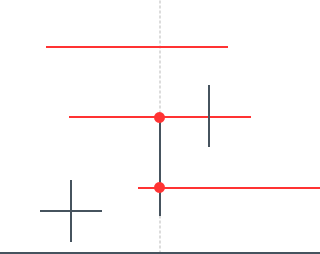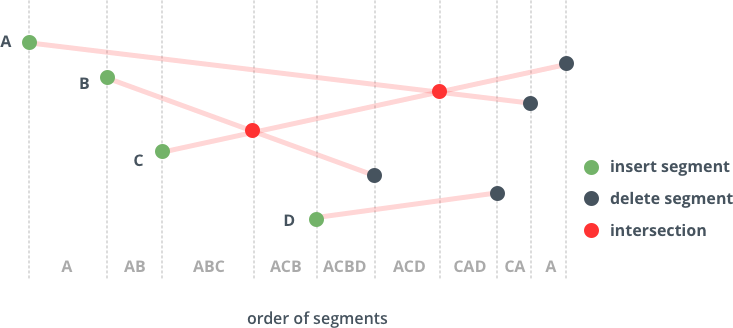Math
Topics:
Line Intersection using Bentley Ottmann Algorithm
• Number Theory
• Combinatorics
• Geometry

# Line Intersection using Bentley Ottmann Algorithm

• Tutorial

Pre-requisite: Line Sweep Algorithms

Line intersection problem is a problem solved using line sweep technique. First , let us define the problem formally.

Problem: Given a set of N line segments(2*N points), you need to find all intersections between these line segments.
So, the first thing that comes to mind is a naive approach to check all pairs of segments whether they intersect or not. But you know that's not a good way as it includes unnecessary computation if we have less intersections. Secondly, it would give intersections in unsorted order. So, we need some alternate approach to solve this problem.
We can solve this problem using line sweep technique.But before going to this problem, first let us consider only horizontal and vertical line segments.

Problem: Given N horizontal and vertical line segments, we need to find all the intersections of horizontal and vertical line segments. Here, we won't consider coincident endpoints to intersect.
Approach: Going on with our notion of events and active set, lets first define them for this problem. Here, we will consider three types of events: start of horizontal line segment, end of horizontal line segment and vertical line segment. Our active set contains all horizontal line segments cut by sweep line (sorted by y coordinate).The dotted line is the sweep line, the black lines are the given horizontal and vertical lines, the red lines are the horizontal lines intersecting the sweep line at any instant.
Our algorithm is as follows:
1. When we hit starting point of horizontal line segment, we insert the line (in our implementation, we will insert the starting point) in our set.
2. When we hit end point of horizontal line segment, we remove the line segment (starting point of line segment in the implementation) from the set.
3. When we hit a vertical line, we check for all the line segments in the set which lie between the starting and ending y coordinate of vertical line segment, i.e., if vertical line segment is indicated by (x1,y1) and (x1,y2), we check for horizontal line segments lying in the range (y1,y2).

#define x second
#define y first
typedef pair<int,int >point;
struct event
{
point p1,p2;
int type;
event() {};
event(point p1,point p2, int type) : p1(p1), p2(p2),type(type) {};  //initialization of event
};
int n,e;
event events[MAX];
bool compare(event a, event b)
{
return a.p1.x<b.p1.x;
}
set<point >s;
void hv_intersection()
{
for (int i=0;i<e;++i)
{
event c = events[i];
if (c.type==0) s.insert(c.p1);//insert starting point of line segment into set
else if (c.type==1) s.erase(c.p2);//remove starting point of line segment from set, equivalent to removing line segment
else
{
for (typeof(s.begin()) it=s.lower_bound(make_pair(c.p1.y,-1));it!=s.end() && it->y<=c.p2.y; it++) // Range search
printf("%d, %d\n", events[i].p1.x, it->y);//intersections
}
}
}
int main ()
{
scanf("%d", &n);
int p1x,p1y,p2x,p2y;
for (int i=0;i<n;++i)
{
scanf("%d %d %d %d", &p1x, &p1y,&p2x, &p2y);
if(p1x==p2x)                //if vertical line, one event with type=2
{
events[e++]=event(make_pair(p1y,p1x),make_pair(p2y,p2x),2);
}
else                    //if horizontal line, two events one for starting point and one for ending point
{
//store both starting points and ending points
events[e++]=event(make_pair(p1y,p1x),make_pair(p2y,p2x),0);
//store both ending and starting points, note the order in the second, this is because we sort on p1, so ending points first, then we remove a line when we hit its ending point , so we need its starting point for removal of line
events[e++]=event(make_pair(p2y,p2x),make_pair(p1y,p1x),1);
}
}
sort(events, events+e,compare);//on x coordinate
hv_intersection();
return 0;
}

Complexity Analysis: All the operations on events (insert,erase, lower_bound) take $O(log N)$ time and the inner loop runs k times where k is the number of intersections. So, in all the complexity of above algorithm is $O(N log N + k)$.
So, the next question that comes to mind is what if k is $O(N^2)$, so in that case our algorithm works slow. It is right but think what if we have $O(N)$ intersections, then we get a considerable speedup. Secondly, what if we need only the number of intersections and not the intersections itself. Then we can find the number of intersections in $O(N log N)$ time by using binary tree structure(by storing the size of subtree in the root of the subtree ).

Let's get back to our problem where the lines are not necessarily vertical or horizontal.What to do in that case?
First, let us list down the assumptions in the algorithm:
1. There are no vertical segments.
2. No two segments intersect at their endpoints.
3. No three (or more) segments have a common intersection.
4. All endpoints of the segments and all intersection points have different x-coordinates.
5. No two segments overlap.

Key Observations:
1. For two lines to intersect, they must be adjacent to each other. So, we will check only for adjacent lines whether they intersect or not.
2. When two line segments intersect, they change their places, that is, the line which was below before intersection goes above and the other line goes below.

Before going to the algorithm, first let us define the events and active set.
Events: end points of line segments, intersection points.(We will insert the intersection point as we find them). Here, we would use priority queue as our data structure as presort wouldn't work because of dynamic insertion and deletion of intersection points.Let us denote the priority queue by PQ
At any moment, the active set contains the line segments cut by sweep line , sorted by y coordinate.Let us denote this active set by SL.
Pseudo Code:

    Initialize PQ = all segment endpoints;
Initialize SL to be empty;
Initialize output intersection list IL to be empty;

While (PQ is nonempty) {
Let E = the next event from PQ;
If (E is a left endpoint) {
Let segE = segment of E;
Let segA = the segment Above segE in SL;
Let segB = the segment Below segE in SL;
If (I = Intersect( segB with segA) exists)
Delete I from PQ;
If (I = Intersect( segE with segA) exists)
Insert I into PQ;
If (I = Intersect( segE with segB) exists)
Insert I into PQ;
}
Else If (E is a right endpoint) {
Let segE = segment of E;
Let segA = the segment Above segE in SL;
Let segB = the segment Below segE in SL;
Delete segE from SL;
If (I = Intersect( segA with segB) exists)
Insert I into PQ;
}
Else {  // E is an intersection event
Add intersect point of E to the output list IL;
Let segE1 above segE2 be intersecting segments of E in SL;
Swap their positions so that segE2 is now above segE1;
Let segA = the segment above segE2 in SL;
Let segB = the segment below segE1 in SL;
If (I = Intersect( segE1 with segA) exists)
Delete I from PQ;
If (I = Intersect( segE2 with segB) exists)
Delete I from PQ;
If (I = Intersect(segE2 with segA) exists)
Insert I into PQ;
If (I = Intersect(segE1 with segB) exists)
Insert I into PQ;
}
remove E from PQ;
}
return IL;
}

That was Bentley Ottmann Algorithm for finding all intersections when given N line segments.Let's take a look at an image to understand it better.The ordering is the order of SL at the events.
So, let's understand it keeping in mind the key observations we made earlier.
We extract the minimum in PQ and make that our event. So, we know this event may be of a left endpoint, right endpoint or an intersection point.
On encountering a left endpoint, the two line segments which were neighbours earlier remain no longer neghbours as we have inserted a segment between them, so we will remove their intersection point if any from PQ. Now the new neighbours are: segA and segE; segB and segE, so we find their intersections(if any) and insert into PQ .
When we visit a right endpoint, we just delete the segment from SL and the two neighbours of segE become the new neighbours of each other, so we check for their intersection.
When an intersection point is visited, the position of the two line segments change (key observation 2), so we delete the intersections of the previous neighbours and insert the intersections of the new neighbours.
Finally, we print the list IL, remember the list is sorted as we were sweeping the line from left to right and inserting the intersection points accordingly.

Complexity Analysis: In all , we have 2N endpoints and k intersections, so total events would be (2N+k). Now, the operations we are doing are insert, delete, find neighbours and swap , all of these could be done in $O(log N)$ using balanced binary tree. Similarly, for PQ we are using operations insert and delete which can be done in O(log N). Initially, the formation of PQ requires $O(N log N)$.So , the overall complexity would be $O(N log N + (2N+k) log N)$=$O(Nlog N+k log N)$.
Now, if k is $O(N^2)$, this goes slower than our brute force algorithm but this has got a considerable speedup if the k is O(N). There are better algorithms than this one for line intersections, but we prefer this because of its simplicity.

On a conclusive note of this tutorial , I would like to discuss one more problem, that is , finding if any two line segments intersect in given N line segments. The same algorithm we applied above can be used with some changes as:
1. We won't consider any intersection event, that means we can presort instead of using PQ.
2. When we encounter any intersection , we return true.
That was line sweep's one more application. Now,try on the attached problem.

Contributed by: Shubham Gupta

?